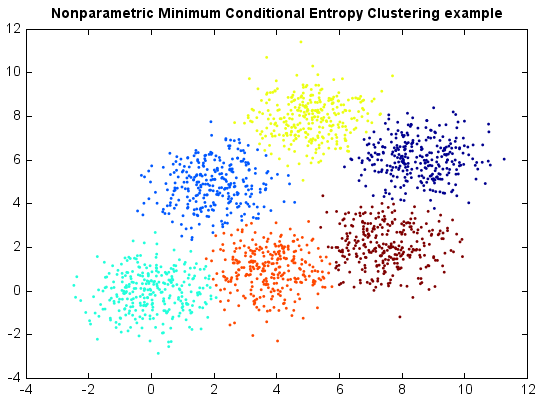# Minimum Entropy Clustering¶

In this algorithm, the clustering criterion is based on the conditional entropy H(C | x), where C is the cluster label and x is an observation. According to Fano’s inequality, we can estimate C with a low probability of error only if the conditional entropy H(C | x) is small. Minimum Entropy Clustering (MEC) also generalizes the criterion by replacing Shannon’s entropy with Havrda-Charvat’s structural α-entropy. Interestingly, the minimum entropy criterion based on structural α-entropy is equal to the probability error of the nearest neighbor method when α = 2. To estimate p(C | x), MEC employs Parzen density estimation, a nonparametric approach.

This method performs very well especially when the exact number of clusters is unknown. The method can also correctly reveal the structure of data and effectively identify outliers simultaneously.

```from miml import datasets
from miml.cluster import MEC

fn = os.path.join(datasets.get_data_home(), 'clustering', 'gaussian',
'six.txt')